# Cuboid

Cuboid with edge a=6 cm and body diagonal u=31 cm has volume V=900 cm3. Calculate the length of the other edges.

Result

b =  30 cm
c =  5 cm

#### Solution:

Checkout calculation with our calculator of quadratic equations.

$c=c_{2}=5 \ \text{cm} \ \\ \ \\ \text{ Correctness test: } \ \\ d=\sqrt{ a^2+b^2+c^2 }=\sqrt{ 6^2+30^2+5^2 }=31 \ \\ d=u \ \\ V_{2}=a \cdot \ b \cdot \ c=6 \cdot \ 30 \cdot \ 5=900 \ \text{cm}^3 \ \\ V_{2}=V$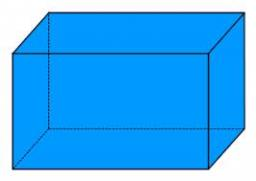Our examples were largely sent or created by pupils and students themselves. Therefore, we would be pleased if you could send us any errors you found, spelling mistakes, or rephasing the example. Thank you!

Leave us a comment of this math problem and its solution (i.e. if it is still somewhat unclear...):Be the first to comment!Tips to related online calculators
Looking for help with calculating roots of a quadratic equation?
Do you have a system of equations and looking for calculator system of linear equations?
Do you want to convert length units?
Tip: Our volume units converter will help you with the conversion of volume units.
Pythagorean theorem is the base for the right triangle calculator.

## Next similar math problems:

1. Block or cuboidThe wall diagonals of the block have sizes of √29cm, √34cm, √13cm. Calculate the surface and volume of the block.
2. Three faces of a cuboidThe diagonal of three faces of a cuboid are 13,√281 and 20 units. Then the total surface area of the cuboid is.
3. At theAt the presentation of the travelers came three times as many men than women. When eight men left with their partners, there were five times more men than women at the presentation. How many were men and women originally?
4. Water current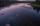John swims upstream. After a while, he passes the bottle, from that moment he floats for 20 minutes in the same direction. He then turns around and swims back, and from the first meeting with the bottle, he sails 2 kilometers before he reaches the bottle.
5. Guppies for sale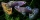Paul had a bowl of guppies for sale. Four customers were milling around the store. 1. Rod told paul - I'll take half the guppies in the bowl, plus had a guppy. 2. Heather said - I'll take half of what you have, plus half a guppy. The third customer, Na
6. Wines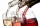Eleven liters of white wine and eight liters of red wine cost a total of 1315 kc. 1 liter of white wine was 10 kc cheaper than a liter of red wine. How much is 1 liter of white and how much red wine?
7. In the classroomThere are 30 boys and a few girls in the class. In the six months, 28 boys and all girls benefited, which was 95% of all pupils. How many pupils are there in the classroom?
8. Bent scaleMonica weighed 52 kg. Sara 54 kg. Together they weighed 111 kg. They noticed that the weight on the scale was bent. How much did they really weigh?
9. Vector perpendicularFind the vector a = (2, y, z) so that a⊥ b and a ⊥ c where b = (-1, 4, 2) and c = (3, -3, -1)
10. The touristThe tourist wanted to walk the route 16 km at a specific time. He, therefore, came out at the necessary constant speed. After a 4 km walk, however, he fell unplanned into the lake, where he almost drowned. It took him 20 minutes to get to the shore and re
11. Vector equationLet’s v = (1, 2, 1), u = (0, -1, 3) and w = (1, 0, 7) . Solve the vector equation c1 v + c2 u + c3 w = 0 for variables c1 c2, c3 and decide weather v, u and w are linear dependent or independent
12. Large familyI have as many brothers as sisters and each my brother has twice as many sisters as brothers. How many children do parents have?Divide number 135 into two additions so that one adds 30 more than 2/5 of the other add. Write the bigger one.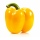The cook was doing meal - in ratio 4: 3: 1 mix tomatoes: pepper: onion. Onions were 5 kg less than peppers. How many kgs of tomatoes did he need to prepare the meal?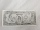Two accounts in the bank, one per year interest 2%, the second 3%. Total interest income 1900 USD. If interest rates were reversed, the yield would be USD 200 higher. What are the amounts on each account?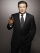Men's shirt has sewn 9 buttons and blouse 3 buttons. Together they sewed 60 pieces and consumed 390 buttons. How many shirts?In the competition, 10 contestants answer five questions, one question per round. Anyone who answers correctly will receive as many points as the number of competitors answered incorrectly in that round. One of the contestants after the contest said: We